AN INTRODUCTION TO ENTROPY

```
```

This page provides a simple, non-mathematical introduction to entropy suitable for students meeting the topic for the first time.

```
```

What is entropy?

At this level, in the past, we have usually just described entropy as a measure of the amount of disorder in a system. A very regular, highly ordered system (diamond, for example) will have a very low entropy. A very disordered system (a mixture of gases at a high temperature, for example) will have a high entropy.

We now expand on this a bit, but luckily not too much! Let's look at this with a couple of thought experiments . . .

Suppose you held a stack of ten coins between your finger and thumb. That's a fairly ordered state for them to be in. And then you dropped them on the floor. Every time you did this, you would get a different random pattern of coins on the floor - arranged just by chance. That is now a disordered system.

Now, it is just imaginable that when you dropped them, by chance they would fall into a neat stack of coins like the one you started with, but the probability of that happening, compared to all the other ways that the coins might fall, is so very, very tiny that you would be totally amazed if it happened.

Technically, entropy applies to disorder in energy terms - not just to disordered arrangements in space. But we often just quickly look at how disordered a system is in space in order to make a judgement about its entropy. A system which is more disordered in space will tend to have more disorder in the way the energy is arranged as well.

Suppose you managed to arrange some gaseous molecules in a container so that they were all exactly evenly spaced and so that they all had exactly the same energy - a fairly ordered state. And then you let them go and do what molecules do - move around, and bump into each other and the walls of the container.

Each collision between two molecules will cause them to change direction, and it will probably speed up one of them, and slow down the other. After a very short time, their arrangement in space will be chaotic, and so will the way energy is shared between them. The faster moving particles have more energy; the slower ones less. The entropy has increased in terms of the more random distribution of the energy.

In essence . . . "a system becomes more stable when its energy is spread out in a more disordered state".

That is really all you need to know. If you look in textbooks or on the web, you will find explanations of increasing difficulty - some very scary indeed! Don't waste time on these at this level.

```
```

Entropy changes during physical changes

Changes of state

This includes solid to liquid, liquid to gas and solid to aqueous solution.

Entropy is given the symbol S, and standard entropy (measured at 298 K and a pressure of 1 bar) is given the symbol S°. You might find the pressure quoted as 1 atmosphere rather than 1 bar in older sources. Don't worry about it - they are nearly the same. 1 bar is 100 kPa; 1 atmosphere is 101.325 kPa. Use whatever units the examiners give you.

Here are some standard entropies for a few solids, all with the units J K-1mol-1:

 carbon (as diamond) 2.4 calcium 41.4 calcium fluoride 68.9 calcium carbonate 92.9

These all have low entropies because they are highly ordered solids, but notice that the entropy usually increases as the solid gets more complicated.

```
```

What happens during change of state? The following figures are for the standard entropy of water in different states.

 ice (approximate value) 48 liquid water 69.9 steam 189

The entropy increases as the molecules become more disordered as you go from solid to liquid to gas.

Notice that there isn't very big jump in entropy when ice turns to water. That's because the hydrogen bonding between the liquid molecules imposes a fair amount of order on them even in the liquid.

. . . and for benzene:

 liquid benzene 173 benzene vapour 269

Notice that the benzene values are bigger than those of water-steam. This is because benzene is a more complicated molecule. There are more ways of arranging the energy of the molecule in a disordered way over bigger molecules than smaller ones.

```
```

What happens when an ionic solid dissolves in water?

The ionic solid is highly ordered, and so has a relatively low entropy. Pure liquid water also has a certain amount of order as explained above. But when the solid dissolves in water, the whole system becomes highly disordered as the crystal breaks up and the ions find their way between the water molecules. Entropy increases.

Note:  Notice that I have taken a short cut here. I have gone straight from an increase in disorder in space and movement to an increase in entropy without specifically thinking about it in energy terms. We often do this at this level. The increase in disorder in space and movement is associated with an increase in disorder in energy terms, but is much easier to imagine.

```
```

Entropy changes when temperature changes

Obviously if increasing the temperature involves a change of state in the material (from solid to liquid or liquid to gas), then you have increased the entropy . . . and we have already looked at that.

Suppose there isn't a change of state.

Think back to having some gas particles in a container with a high degree of disorder both in their spacial arrangement, and in their energies - they are moving at all sorts of different speeds, changing all the time.

If you add more energy by heating the gas, the number of different possibilities for arranging the energy over the molecules increases. And so increasing the temperature increases the entropy of the system.

```
```

Entropy changes in reactions involving at least some gas molecules

Gases have higher entropies than solids or liquids because of their disordered movement. That means that during a reaction in which there is a change in the number of molecules of gas present, entropy will change.

Reactions involving an increase in the number of gas molecules

For example, the effect of heat on calcium carbonate, or the reaction between a carbonate and an acid: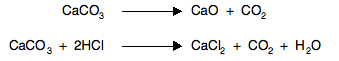In both cases, there are no gases on the left-hand side of the equation, but carbon dioxide appears on the right. Entropy will increase during such a reaction, because of the increased disorder.

Reactions involving a decrease in the number of gas molecules

For example, the production of ammonia: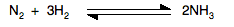In this case, there is a decrease in entropy during the forward reaction because there are fewer gas molecules than you had to start with. That means that there fewer ways of arranging the energy of the system over those molecules, and so entropy decreases.

```
```

Predicting how entropy will change during reactions or other changes

Example 1: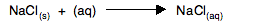This is just a crystalline solid going into solution. The solid is highly ordered; the solution is disordered. There is an increase in entropy.

Example 2: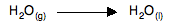The water is changing from the highly disordered gas into a more ordered liquid. The entropy will fall.

Example 3: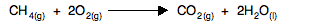There are three moles of gas on the left-hand side of the equation, but only one on the right. The starting materials are more disordered than the products, and so there is a decrease in entropy.

Notice that if the water had been formed as steam, you couldn't easily predict whether there was an increase or a decrease in entropy, because there would be three moles of gas on each side.

Example 4:The presence of the five moles of liquid water on the left-hand side means that there will be far more disorder before the change than there is in the products. The copper(II) sulphate crystals formed will be very ordered. Entropy will decrease.

```
```
 Questions to test your understanding Because this is all covered in detail in my calculations book I shan't be setting any questions throughout this section on entropy and free energy
```
```

Where would you like to go now?

To the entropy and free energy menu . . .

To the Physical Chemistry menu . . .

To Main Menu . . .

```
```